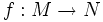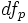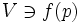# Local immersion theorem

Let$M$ and$N$ be differential manifolds of dimensions$m < n$. Let$f:M \to N$ be a differentiable map between them. Let$p \in M$ be a point such that$f$ is an immersion at$p$ -- in other words, the induced map$df_p$ is injective. Then, there exists a neighbourhood$U \ni p$ in$M$ and$V \ni f(p)$ in$N$, and a choice of coordinate charts, such that the restriction of$f$ to$U$, viewed using those coordinate charts, is a map of the form:$(x_1,x_2,\ldots,x_m) \mapsto (x_1, x_2, \ldots, x_m, 0, 0, \ldots, 0)$